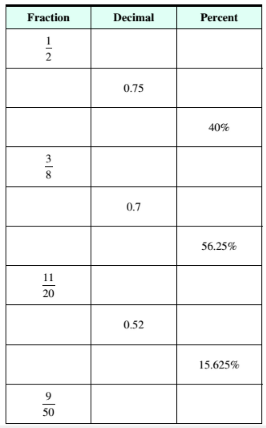Chapter 9.3, Problem 12ESMathematical Excursions (MindTap C...

4th Edition
Richard N. Aufmann + 3 others
ISBN: 9781305965584

Solutions

Chapter
SectionMathematical Excursions (MindTap C...

4th Edition
Richard N. Aufmann + 3 others
ISBN: 9781305965584
Textbook Problem

Complete the table of equivalent fractions, decimals, and percent’s.To determine

To calculate: The decimal and percent for the fraction 950 .

Explanation

Given Information:

Fraction, 950

Formula used:

To write fraction as decimal, perform division of the numerator by the denominator.

Calculation:

Consider the fraction,

950

Perform division of numerator by the denominator to write fraction 950 as decimal,

950=0

Still sussing out bartleby?

Check out a sample textbook solution.

See a sample solution

The Solution to Your Study Problems

Bartleby provides explanations to thousands of textbook problems written by our experts, many with advanced degrees!

Get Started